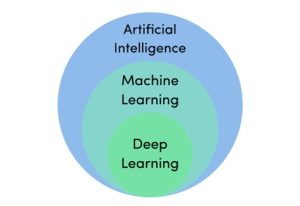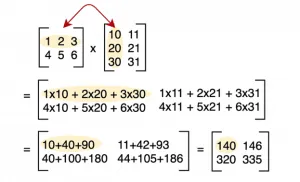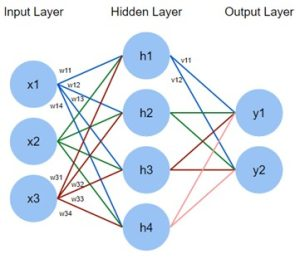# Matrix Operations for Deep Learning and Machine Learning

Matrix operations like addition, subtraction, multiplication, and division are the most important step in deep learning and machine learning. The person who wants to become in such fields must have all the tricks of such operation in the bag in order to get a better understanding of these fields. Matrix operations are not only important in regular multiplications, additions, etc but also important when the coding part of deep learning arrives. So, a scientist, researcher, or engineer must have a better understanding of all these operations so that no confusion occurs at that time.

#### Deep Learning and Machine Learning

Before preceding further, it is important to learn the basic differences between these two fields and how they affect the way of research and technology. In machine learning, there is an algorithm that is given initially to the system with the help of which the system can learn, extract useful data, and make informed decisions based on learning. On the other hand, in deep learning, there are layers of algorithms that make artificial neural networks. In deep learning, the system is capable of taking decisions without informing the outside environment. Deep learning is a subset of machine learning, and both of these technologies come under the broad field of artificial intelligence.Figure 1. A visual description: Deep Learning is a complex subset of Machine learning. Whereas Machine Learning is a part of Broad Artificial Intelligence 

#### What is Matrix Operation?

Matrix operations are basically the three basic algebraic expressions which are addition, subtraction, and multiplication of matrix. While dealing with matrices the scientist, researcher, or the engineer must have know-how about all these operations in order to make accurate results, implement those results, and execute the plans which come as a result of these operations. Then there is also a subset of these operations like column operations and row operations. The multiplication of matrices can also be scalar (in which a single scalar number can be multiplied with each the entry of the matrix) and matrix multiplication (in which each element of the nth row of the first matrix is multiplied with each element of the nth column of the second matrix and added up in the end in order to get a single entry in the resultant matrix)  as explained in the figure below.Figure 2. An understanding of Matrix Multiplication (A common Matrix Operation) 

#### Importance of Matrix in Machine Learning and Deep Learning

While dealing with artificial intelligence and its subset fields the researcher may have come across different matrices for this purpose all the basic, mediocre, and complex operations related to matrix operations should have been understood by the person himself so that there will be no confusion during the coding part of deep learning or machine learning. Each entry of a matrix represents data, sometimes these data can be represented in the form of a mathematical equation but to represent data in the form of a matrix is much easier and more concise. Following are the few advantages of matrices over other complex algebraic equations in deep learning.

• Deep learning and machine learning are confusing itself if we use algebraic equations for solving such problems then it would be more confusing as in a matrix all the data is confined in a bracket and all the operations can be performed inside a matrix to obtain fruitful results.
• With the help of matrices, the description of the algorithm from the developer end is comparatively easy while dealing with regular algebraic equations. 
• In the figure given below, we have observed there are different layers of a complex neural network like the input layer, hidden layer, and output layer. Each layer has multiple branches, and each branch has multiple weights assigned to them. So, it is a common observation as shown in the figure that by applying regular mathematics to these branches, layers, and weight it will be very difficult for the user, developer, and even the code to understand the scenario. That is the reason a matrix can be used here, and with its regular matrix operations training of the system can be done and a robust system can be developed.Figure 3. A representation of a Complex Neural Network. 

• In deep learning and machine, learning problems approximation played a very important role due to the involvement of fuzzy logic type tools. So, with the help of matrices, better approximations can be obtained. The calculations of deep learning and machine learning are also complex in nature, so with the help of matrix operation, one can approximate the calculations and easily train the system. 
• Matrix operations are faster as compared to regular algebraic expressions as in matrix operation many entries multiply, add, and subtract from each other simultaneously giving us better results in lesser time. Hence, in machine learning and deep learning matrix operations are preferred not only for the theoretical part but also for the coding part it is important. 

#### Example of Matrix Operation from Literature

In an article, there are a lot of practical implementations of matrix operations. In the figure given below as we can observe there are a large number of inputs, outputs, layers, and parameters. While solving these with the help of regular algebra, it will be very time-consuming and complex as well. In this article, the author has observed the time of a batch of iterations using the approximation technique from the matrices and with the help of further approximation other results can be determined, and hence a lot of time can be saved using this simple approximation. They have used the following different techniques in order to minimize the time and decrease the complexity of the system to obtain better results. 

• They have used “Caffe Train” command for training purposes.
• CNTK algorithm to find the initial time of input and output
• Tensor flow has been used here to find the average time in which each iteration has been done
• Torch has also been used here. Torch has the same objectives as tensor flow, but Torch is basically used here for performance evaluation.Figure 4. A Sample of Iteration from Literature 

Also Read: Are Machine Learning and Data Science the same?

### Get In Touch

Scale up your remote team and execute projects on time

Kickstart Your Digital Transformation Journey Today

## We would love to hear from you

250+ successful projects delivered by a team of 90+ passionate engineers.

Reach us at: